# Open End Correction

The theme of this lecture is open end correction.
This is a term you will often see when trying to solve air-column problems.

There are two types of air-column problems: those that take into account the effect of open end correction and those that ignore it.
I will illustrate the difference in approach to these two types of problems through examples!

## When open end correction is negligible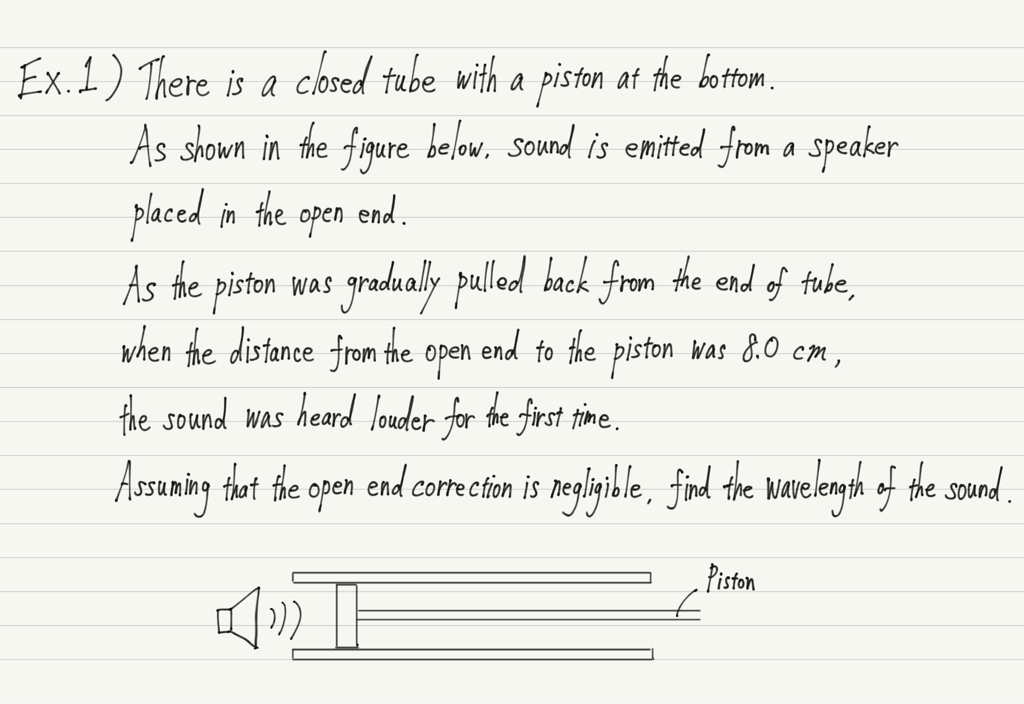First of all, it is not necessary to determine for yourself whether the open end correction is negligible or not.
If the text says “open end correction is ignored”, then it is ignored; if it says nothing, then the open end correction is usually taken into account.

If you ignore it, there is no need to think about anything special, so just write down the form of the fundamental vibration as we did for the air-column and find the equation relating wavelength to the length of the tube.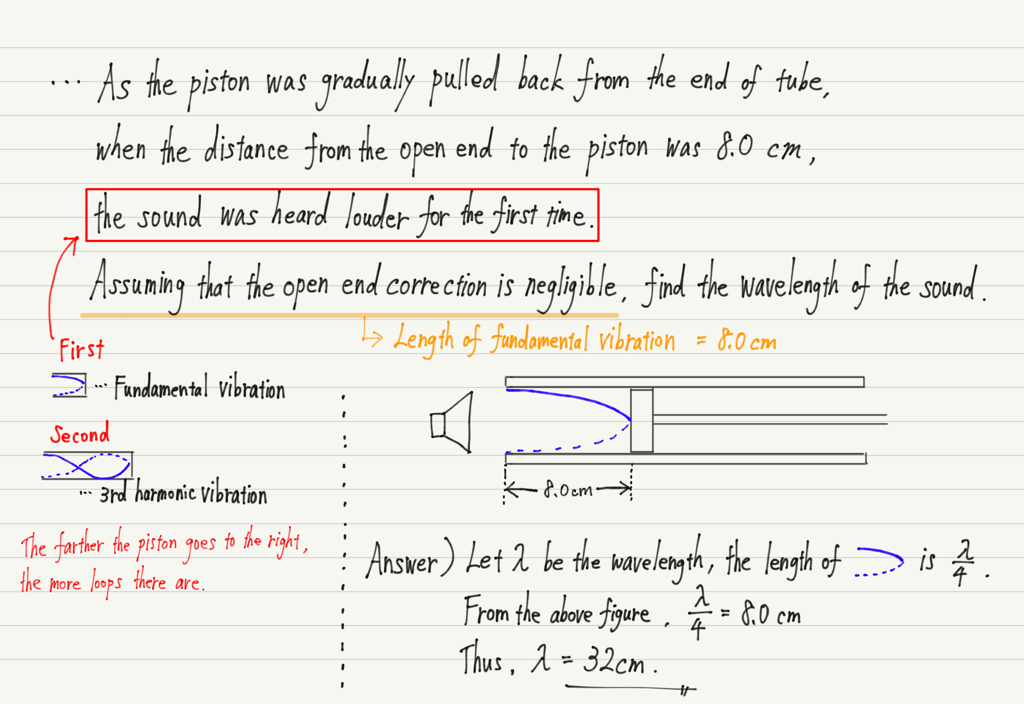## When open end correction is not negligible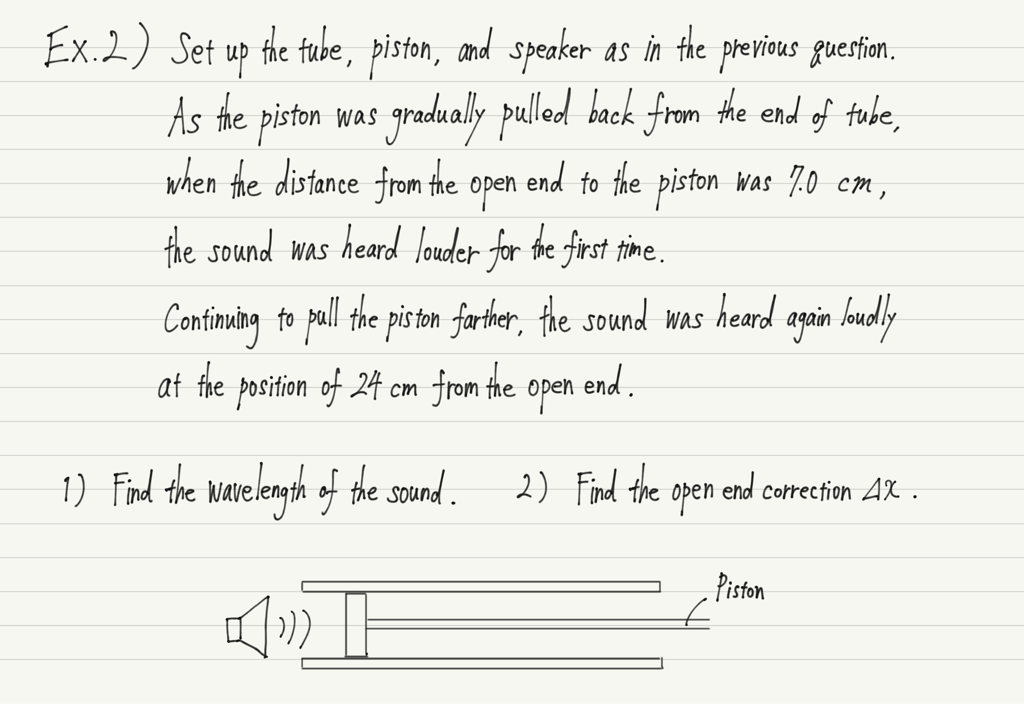In this case, the solution of the previous question cannot be used.
If you know what an open end correction is, you will understand why.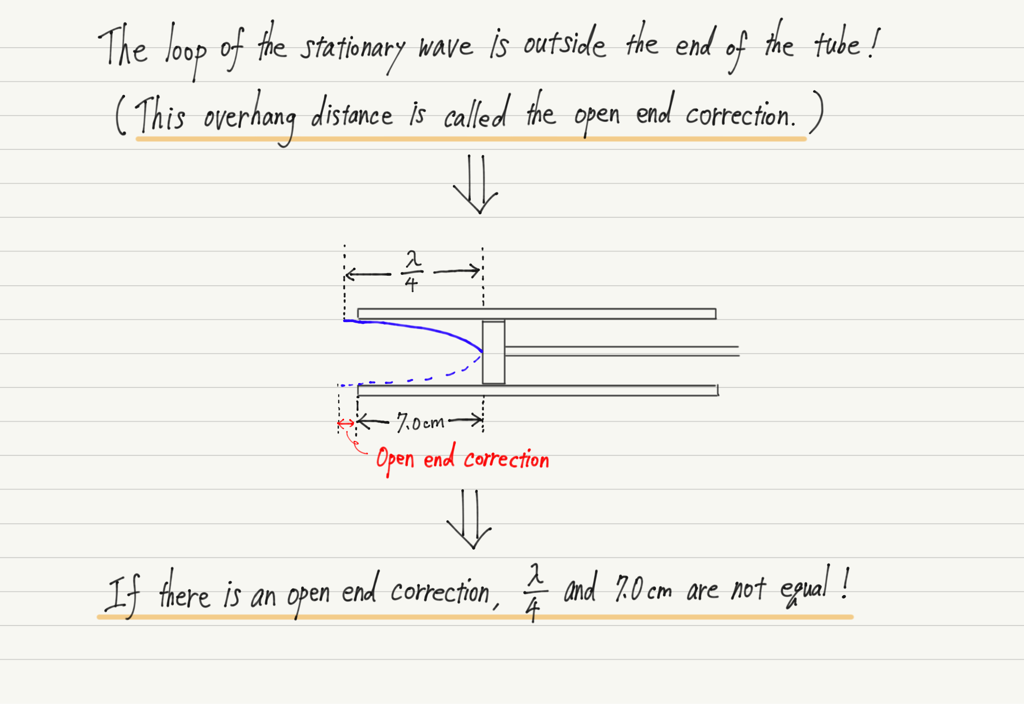Now, what should we do in such a case?

As the name implies, open end correction is a phenomenon that occurs at the end of a tube.
In other words, if you use the far side of the tube instead of the end, your calculations will be unaffected by the open end correction!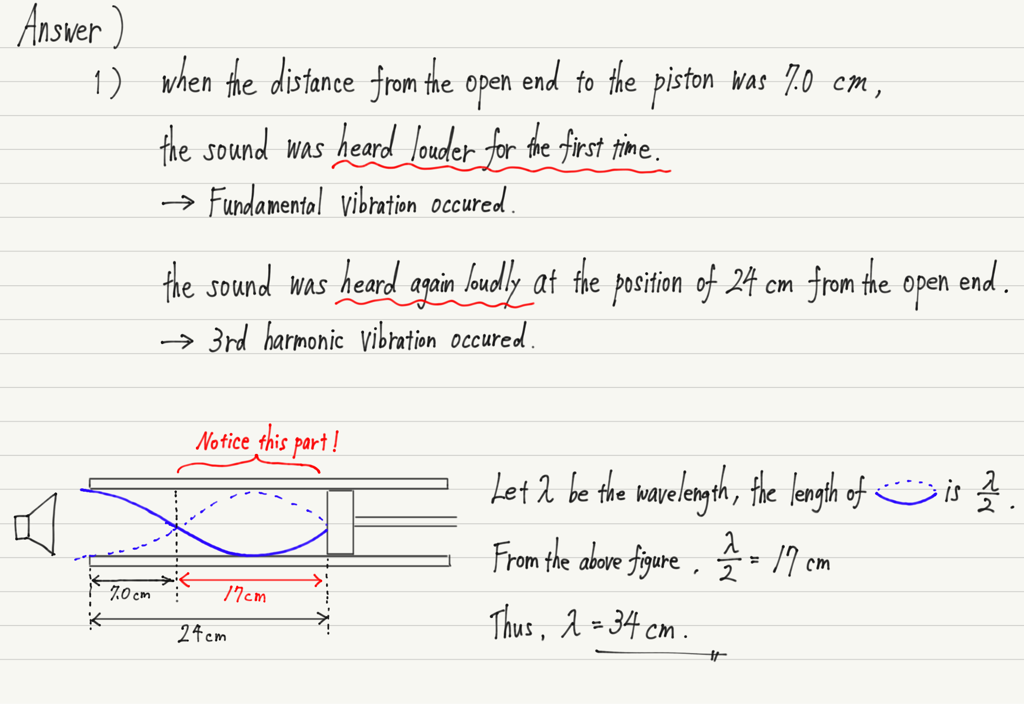Next, 2), the calculation of the open end correction, let’s use the end of the tube for this calculation.

The open end correction is easily obtained by drawing a picture.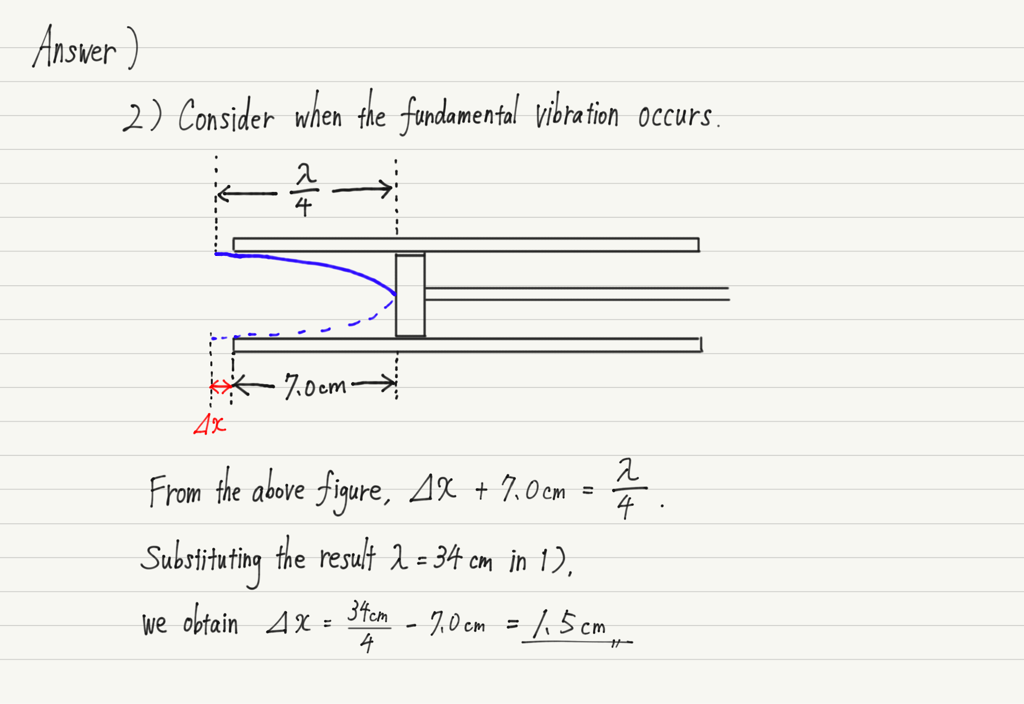## Summary of this lecture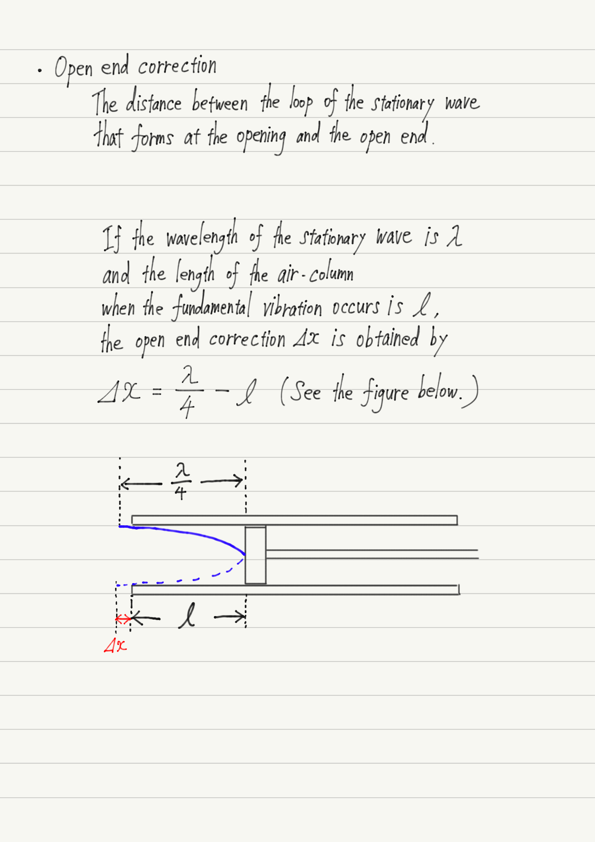error: Content is protected !!